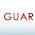## Classifying Iris dataset using Naive Bayes Classifier

The Iris Dataset is a multivariate dataset. It has 5 attributes, the first one is sepal length (Numeric), second is sepal width (Numeric) third one is petal length (Numeric), the fourth one is petal width (Numeric) and the last one is the class itself. We have 150 rows that are equivalent to 150 flowers collected those flowers are divided into the different category. They are similar flowers that are Iris but the different category like Iris-setosa, Iris-versicolor, and Iris-virginica. It is important to know about these patterns because in future if you see similar data we can say that this data belong to the certain pattern. Based on these data, we can predict which kind of the Iris flower does new flower belongs. It is supervised data since we have the class (Nominal).

Data visualization:  As we can see there are three classes. Blue (Iris-setosa), Red (Iris-versicolor), and Sky Blue (Iris-virginica). There are equivalently distributed so during the analysis we don’t have to normalize it. There is same frequency of data of all class. Now let us analyze petal length graph. We can distinctly analyze that if the petal length is from 1 to 2 then it is of Iris-setosa. Similarly, we can analyze others using attributes graph.

Basically, the task is to assign a new data to one or more classes or categories is classification or categorization. Naive Bayes is a simple technique for constructing classifiers: models that assign class labels to problem instances, represented as vectors of feature values, where the class labels are drawn from some finite set. It assumes that the value of a particular feature is independent of the value of any other feature, given the class variable. For example, a fruit may be considered to be an apple if it is red, round, and about 10 cm in diameter. A naive Bayes classifier considers each of these features to contribute independently to the probability that this fruit is an apple, regardless of any possible correlations between the color, roundness and diameter features.

Python Code: Using Python weka wrapper for NaiveBayesUpdateable
Result

=== Summary ===
Correctly Classified Instances         144               96      %
Incorrectly Classified Instances         6                4      %
Kappa statistic                                             0.94
Mean absolute error                                     0.0324
Root mean squared error                            0.1495
Relative absolute error                                 7.2883 %
Root relative squared error                          31.7089 %
Total Number of Instances                            150

=== Confusion Matrix ===
a                  b                    c                       <-- classified as
50                0                    0                     |  a = Iris-setosa
0                  48                 2                     |  b = Iris-versicolor
0                 4                     46                  |  c = Iris-virginica

From this Confusion Matrix, we found that 50 of data is Iris-setosa from the above chart. Then it was able to classify 48 separate ways with 2 errors for Iris-versicolor. For Iris-virginica, 46 were rightly classified and 4 of them were an error.

The naive bayes model is comprised of a summary of the data in the training dataset. This summary is then used when making predictions. The summary of the training data collected involves the mean and the standard deviation for each attribute, by class value. For example, if there are two class values and 7 numerical attributes, then we need a mean and standard deviation for each attribute (7) and class value (2) combination that is 14 attribute summaries. These are required when making predictions to calculate the probability of specific attribute values belonging to each class value. We can break the preparation of this summary data down into the following sub-tasks:
1.       Separate Data By Class
2.       Calculate Mean
3.       Calculate Standard Deviation
4.       Summarize Dataset
5.       Summarize Attributes By Class

We are now ready to make predictions using the summaries prepared from our training data. Making predictions involves calculating the probability that a given data instance belongs to each class, then selecting the class with the largest probability of the prediction. We can divide this part into the following tasks:
1.       Calculate Gaussian Probability Density Function
2.       Calculate Class Probabilities
3.       Make a Prediction
4.       Estimate Accuracy

References
https://github.com/fracpete/python-weka-wrapper

1.Great Article… I love to read your articles because your writing style is too good, its is very very helpful for all of us and I never get bored while reading your article because, they are becomes a more and more interesting from the starting lines until the end.

selenium training in electronic city | selenium training in electronic city | Selenium Training in Chennai | Selenium online Training | Selenium Training in Pune | Selenium Training in Bangalore

1.2.The best gambling establishment on the Internet best online casino here Win with us.

3.Что бы диоды не перегорали, нужно использовать профиль для светодиодов для лучшего теплоотвода что я обычно и делаю)

4.Such a great information for blogger iam a professional blogger thanks…

Start your journey with Best SAP FICO Course and get hands-on Experience with 100% Placement assistance from experts Trainers @Softgen Infotech Located in BTM Layout Bangalore.

5.The student will need to be convinced that what he is doing is good in order to complete the course as well and as quickly as he can. artificial intelligence course in hyderabad